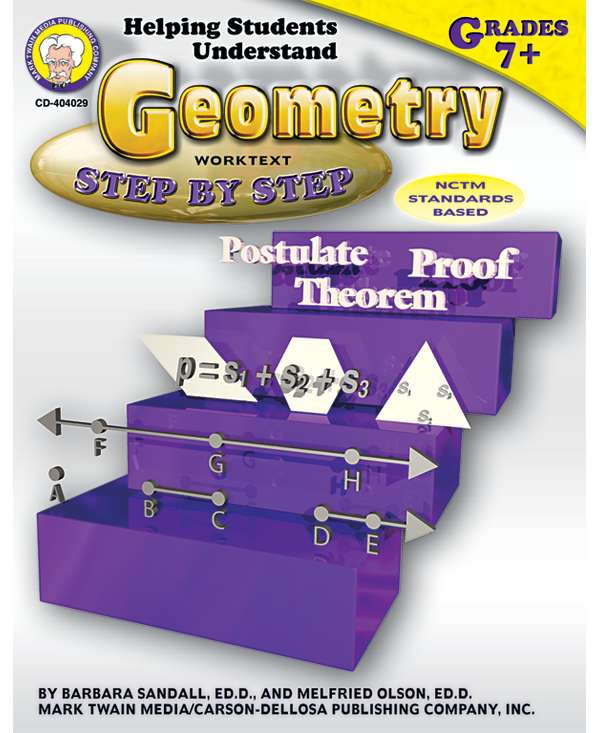•# Helping Students Understand Geometry Step By Step

Product Number: CD-404029
\$13.99
Help students in grades 7 and up transition from math to geometry using Helping Students Understand Geometry.This 128-page ma...
Quantity

Help students in grades 7 and up transition from math to geometry using Helping Students Understand Geometry.

This 128-page math resource book includes:

• step-by-step instructions with examples
• practice problems using the concepts
• real-life applications
• a list of symbols and terms
• tips

The book supports NCTM standards and includes topics such as:

• coordinates
• angles
• patterns and reasoning
• triangles
• polygons and quadrilaterals
• circles

Features: